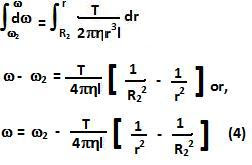## Wednesday, July 8, 2009

### Irodov Problem 1.333Let the viscous torque opposing the rotation of the cylinder be T. The viscous force acting at a distance r from the axis would be F(r)=T/r. Let the length of the cylinder be l, then the viscous stress at a distance r would be,The outer cylinder rotates atand is located at radius R2. So from (3) we have,The angular velocity of the inner cylinder is 0 at radius R1 hence from (4) we have,Also from (5) it is clear that the torque per unit length due to viscous forces is,